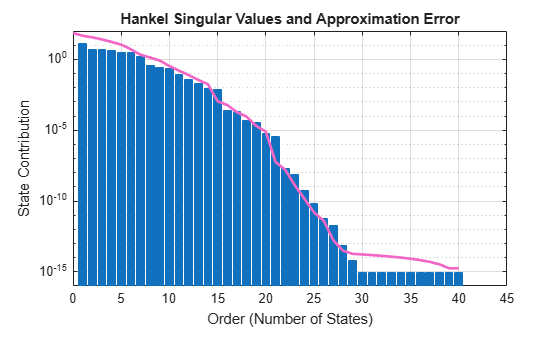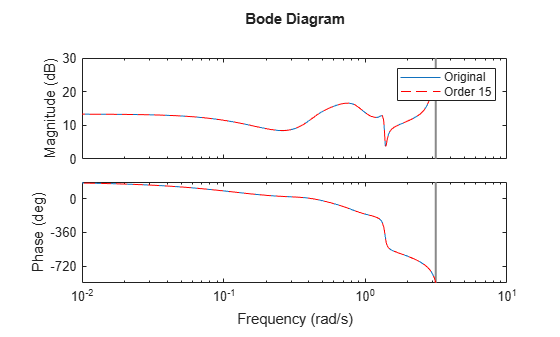# reducespec

Create model order reduction specifications

Since R2023b

## Syntax

``R = reducespec(sys,method)``

## Description

The `reducespec` function is the entry point for model order reduction workflows in Control System Toolbox™ and Robust Control Toolbox™ software. Use this function to create a model order reduction (MOR) task based on the model type and selected method.

For information on configuring model order reduction tasks, see the object corresponding to your model type and selected algorithm. For details on how to select orders and obtain reduced-order models, see the corresponding `view` and `getrom` functions.

Model TypeAlgorithmObjectObject Functions
Linear time-invariant (LTI)Balanced truncation`BalancedTruncation`

Balanced truncation of normalized coprime factors (NCF)

`NCFBalancedTruncation`
Modal truncation`ModalTruncation`
Sparse LTIBalanced truncation`SparseBalancedTruncation`
Modal truncation`SparseModalTruncation`

This function creates only the MOR specification object and does not perform any computation. This allows you to properly configure options before you run the MOR algorithm, which can be computationally expensive in the case of sparse models.

Tip

For the full workflow, see Task-Based Model Order Reduction Workflow.

example

````R = reducespec(sys,method)` creates a model order reduction (MOR) specification object for a dense or sparse linear time-invariant (LTI) model `sys`. `method` is the model order reduction algorithm, specified as one of the following: `"balanced"``"ncf"` (Requires Robust Control Toolbox software)`"modal"` ```

## Examples

collapse all

This example shows the basic workflow for obtaining reduced-order models using the task-based model order reduction workflow.

Generate a random discrete-time state-space model with 40 states.

```rng(0) sys = drss(40);```

Create a balanced truncation model-order reduction task using `reducespec`.

`R = reducespec(sys,"balanced");`

The function returns a `BalancedTruncation` object.

View the graphical information to help decide the reduced order using the `view` function.

`view(R)`For balanced truncation, `view` plots the state contributions in terms of the Hankel singular values.

Obtain the reduced order model using `getrom`. For this example, select an order 15.

`rsys = getrom(R,Order=15);`

Compare the Bode response of both models.

```bode(sys,rsys,'r--') legend("Original","Order 15")```## Input Arguments

collapse all

Dynamic system model, specified as an ordinary or sparse LTI model.

This model must have a valid state-space representation, such as a `tf`, `ss`, `sparss`, or `mechss` model. For generalized or uncertain state-space models (`genss`, `uss`), the function uses the current value of the model. For identified models (`idss`), the function uses the identified value.

Model order reduction algorithm, specified as one of these strings:

• `"balanced"` — Use the balanced truncation algorithm. This method quantifies the state contributions to the I/O transfer and lets you discard states with weak contribution.

• `"ncf"` — Use the balanced truncation algorithm applied to the normalized coprime factors (NCF) of `sys`. This method requires Robust Control Toolbox software and is available only for ordinary LTI models.

• `"modal"` — Use the modal truncation algorithm. This method computes the system modes (poles) and lets you discard modes based on their location or DC contribution.

## Output Arguments

collapse all

Model order reduction specification object, returned as one of the objects in this table. The type of object depends on the model type and algorithm.

Model Type (`sys`)Algorithm (`method`)Object (`R`)
LTI`"balanced"``BalancedTruncation`
`"ncf"``NCFBalancedTruncation`
`"modal"``ModalTruncation`
Sparse LTI`"balanced"``SparseBalancedTruncation`
`"modal"``SparseModalTruncation`

## Limitations

• Sparse modal truncation is currently limited to first-order models with A = AT and E = ET definite, or second-order models with K = KT, M = MT definite, and Rayleigh-type damping.

• Sparse balanced truncation is currently limited to continuous-time models.

 Benner, Peter, Jing-Rebecca Li, and Thilo Penzl. “Numerical Solution of Large-Scale Lyapunov Equations, Riccati Equations, and Linear-Quadratic Optimal Control Problems.” Numerical Linear Algebra with Applications 15, no. 9 (November 2008): 755–77. https://doi.org/10.1002/nla.622.

 Benner, Peter, Martin Köhler, and Jens Saak. “Matrix Equations, Sparse Solvers: M-M.E.S.S.-2.0.1—Philosophy, Features, and Application for (Parametric) Model Order Reduction.” In Model Reduction of Complex Dynamical Systems, edited by Peter Benner, Tobias Breiten, Heike Faßbender, Michael Hinze, Tatjana Stykel, and Ralf Zimmermann, 171:369–92. Cham: Springer International Publishing, 2021. https://doi.org/10.1007/978-3-030-72983-7_18.

 Varga, A. “Balancing Free Square-Root Algorithm for Computing Singular Perturbation Approximations.” In  Proceedings of the 30th IEEE Conference on Decision and Control, 1062–65. Brighton, UK: IEEE, 1991. https://doi.org/10.1109/CDC.1991.261486.

 Green, M. “A Relative Error Bound for Balanced Stochastic Truncation.” IEEE Transactions on Automatic Control 33, no. 10 (October 1988): 961–65. https://doi.org/10.1109/9.7255.